# Java Program to Find ASCII Value of a character

In this tutorial you will learn about the Java Program to Find ASCII Value of a character and its application with practical example.

## Java program to Find ASCII Value of a Character

In this tutorial, we will learn to create a Java program that will find the ASCII Value of a Character in Java programming.

## Prerequisites

Before starting with this tutorial, we assume that you are the best aware of the following Java programming topics:

• Operators in Java Programming.
• Basic Input and Output function in Java Programming.
• Basic Java Programming.

## Program to Find ASCII Value of a Character:-

American Standard Code For Information Interchange stands for ASCII. The ASCII is an encoded format. In Java programming, we can take the input from the user in character format. In today’s program, we will take input in character format. Secondly, we will find the ASCII Value of a Character in the input with the help of a small program.

## Output:-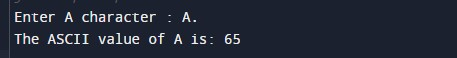In the above program, we have first initialized the required variable.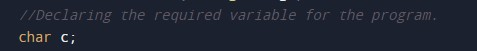• c = it will hold the character value from the user.

Taking input characters from the user for the program.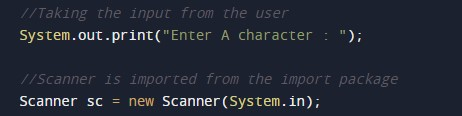Finding the ASCII value of the character from the code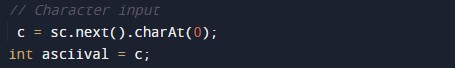Printing the ASCII value of the character.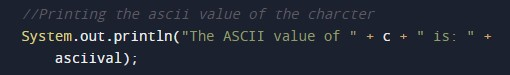In this tutorial we have learn about the Java Program to Find ASCII Value of a character and its application with practical example. I hope you will like this tutorial.